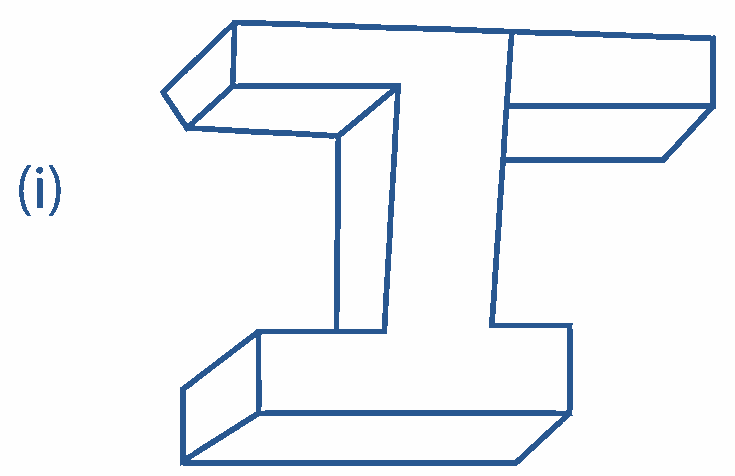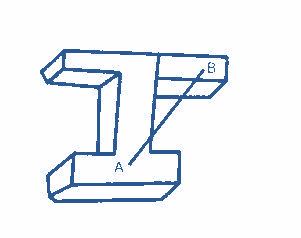The following figure is a

# The following figure is a1. A
Convex polyhedron
2. B
Concave polyhedron
3. C
regular polyhedron
4. D
polygon

Fill Out the Form for Expert Academic Guidance!l

+91

Live ClassesBooksTest SeriesSelf Learning

Verify OTP Code (required)

### Solution:

The given figure isWe know that convex polyhedron is one in which the line segment connecting on the surface of a polyhedron totally sits within or on the polyhedron. Otherwise, it is a concave polyhedron.
In the given figure, let us consider two points A & B.
Join the points AB. We get a line segment AB.The line AB is not totally contained within or on the polyhedron.
So, it is a concave polyhedron
The correct option is (2).

## Related content

 Difference Between Mass and Weight Differences & Comparisons Articles in Physics Important Topic of Physics: Reynolds Number Distance Speed Time Formula Refractive Index Formula Mass Formula Electric Current Formula Electric Power Formula Resistivity Formula Weight Formula+91

Live ClassesBooksTest SeriesSelf Learning

Verify OTP Code (required)